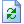﻿ Bayesian estimation with user-defined estimands (custom estimands)

# Bayesian estimation with user-defined estimands (custom estimands)

This video (16 minutes and 53 seconds long) shows how to perform Bayesian estimation with user-defined estimands (also called custom estimands). The example shows how to estimate:

• an indirect effect
• a direct effect
• the difference between the indirect effect and the direct effect
• the probability that the indirect effect is positive
• the probability that the indirect effect exceeds the direct effect

Note: After this video was made, the Snapshot iconwas changed to the Refresh icon